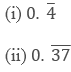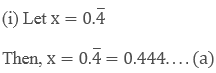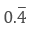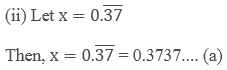×#### Thank you for registering.

One of our academic counsellors will contact you within 1 working day.

Click to Chat

1800-1023-196

+91-120-4616500

CART 0

• 0

MY CART (5)

Use Coupon: CART20 and get 20% off on all online Study Material

ITEM
DETAILS
MRP
DISCOUNT
FINAL PRICE
Total Price: Rs.

There are no items in this cart.
Continue Shopping• Complete JEE Main/Advanced Course and Test Series
• OFFERED PRICE: Rs. 15,900
• View Details

```Chapter 1: Number System Exercise – 1.3

Question: 1

Express each of the following decimals in the form of rational number.

(i)  0.39

(ii) 0.750

(iii) 2.15

(iv) 7.010

(v)  9.90

(vi) 1.0001

Solution:

(i) Given,

0.39 = 39/100

(ii) Given,

0.750 = 750/1000

(iii) Given,

2.15 = 215/100

(iv) Given, 9.101

(iv) Given,

7.010 = 7010/1000

(v) Given,

9.90 = 990/100

(vi) Given,

1.0001 = 10001/10000

Question: 2

Express each of the following decimals in the form of rational number (p/q)Solution:Multiplying both sides of equation (a) by 10, we get,

10x = 4.44.... (b)

Subtracting equation (1) by (2)

9x = 4

x = 4/9

Hence,= x = 4/9Multiplying both sides of equation (a) by 100, we get,

100 x = 37.37....  (b)

Subtracting equation (1) by (2)

99 x = 37

x = 37/99

Hence,= x = 37/99
```### Course Features

• 728 Video Lectures
• Revision Notes
• Previous Year Papers
• Mind Map
• Study Planner
• NCERT Solutions
• Discussion Forum
• Test paper with Video Solution